Friday, October 22, 2021
Home > NSEJS > NSEJS 2019-20 Question Paper With Answer Keys And Solutions

# NSEJS 2019-20 Question Paper With Answer Keys And Solutions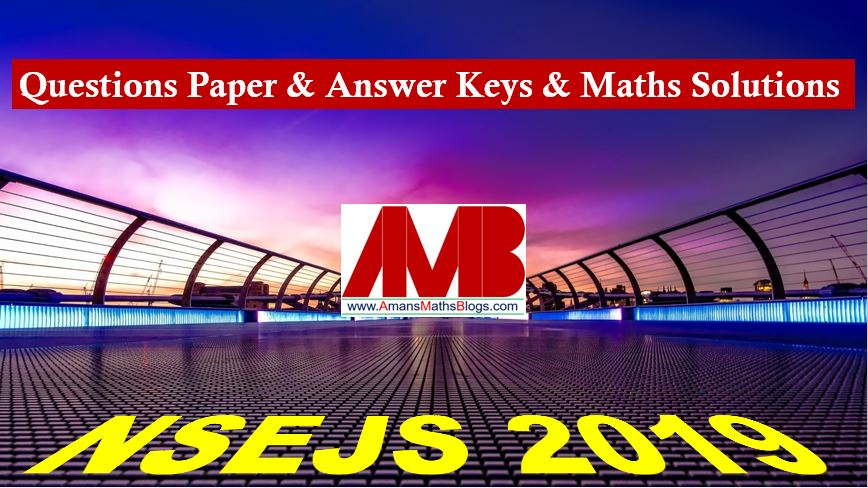# NSEJS 2019-20 Question Paper With Answer Keys And Solutions

NSEJS 2019-20 is an IJSO Stage 1 exam that is conducted by IAPT (Indian Association of Physics Teacher). In 2019-20 session, NSEJS is conducted on 17 November 2019 (Sunday).

Read More : Details of IJSO Stages

## NSEJS Maths Questions Bank EBook

Get NSEJS 2008 to 2018 Maths Questions Bank with Solutions. To get this combo ebook, click below RED button.These questions are solved by me, AMAN RAJ, I am maths faculty of IIT Maths Foundation course and founder of this website AmansMathsBlogs (AMB).

NSEJS
Previous Year Question Papers
2008-092009-102010-112011-122012-13
2013-142014-152015-162016-172017-18
2018-192019-20

## NSEJS 2019-20 Question Paper Code 51

Read More : 129 Maths Short Tricks for NTSE NSEJS JSTSEQues NoAns KeyQues NoAns KeyQues NoAns KeyQues NoAns Key
1D6A11A16C
2B7D12A17A
3C8D13A18D
4C9A14A19C
5D10B15A20B
Ques NoAns KeyQues NoAns KeyQues NoAns KeyQues NoAns Key
21C26B31D36D
22B27C32B37C
23B28D33C38B
24D29D34D39C
25B30C35B40A
Ques NoAns KeyQues NoAns KeyQues NoAns KeyQues NoAns Key
41B46B51A56A
42A47C52A57C
43C48D53A58C
44C49C54B59D
45B50B55B60A
Ques NoAns KeyQues NoAns KeyQues NoAns KeyQues NoAns Key
61C66B71D76C
62A67B72A77A
63B68B73B78A
64D69A74C79A
65C70C75C80D

## NSEJS 2019-20 Solutions MATHS

NSEJS 2019-20 Maths Questions Bank With Solution: Ques No 1

Let α and β be the roots of the equation x2 – 5x + 3 = 0 with α > β. If an = αn – βn for n ≥ 1, then the value of (3a6 + a8)/a7 is

Options:

A. 2

B. 3

C. 4

D. 5

Solution: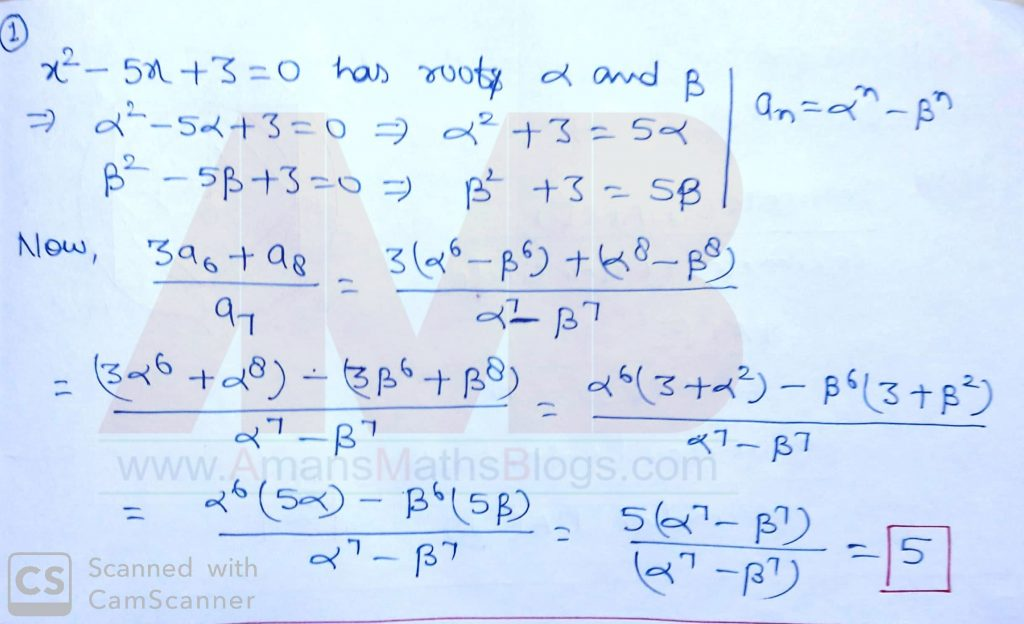NSEJS 2019-20 Maths Question Bank With Solutions: Ques No 2

The number of triplets (x, y, z) such that any one of these numbers is added to the product of the other two, the result is 2, is

Options:

A. 1

B. 2

C. 4

D. Infinite

Solution:NSEJS 2019-20 Maths Question Bank With Solutions: Ques No 3

In a rectangle ABCD, AB = 5 and BC = 3. Points F and G are on the line segment CD so that DF = 1 and GC = 2. Lines AF and BG intersect at E. What is the area of the triangle AEB?

Options:

A. 10 sq. units

B. 15/2 sq. units

C. 25/2 sq units.

D. 20 sq. units.

Solution:NSEJS 2019-20 Maths Question Bank With Solutions: Ques No 4

In the given figure, two concentric circles are shown with centre O. PQRS is a square inscribed in the outer circle. It also circumscribes the inner circle, touching it at the points B, C, D and A. What is the ratio of the perimeter of the outer circle to that of the quadrilateral ABCD?Options:

A. π/4

B. 3π/2

C. π/2

D. π

Solution:NSEJS 2019-20 Maths Questions Bank With Solutions: Ques No 5

How many positive integers N give a remainder 8 when 2008 is divided by N?

Options:

A. 12

B. 13

C. 14

D. 15

Solution: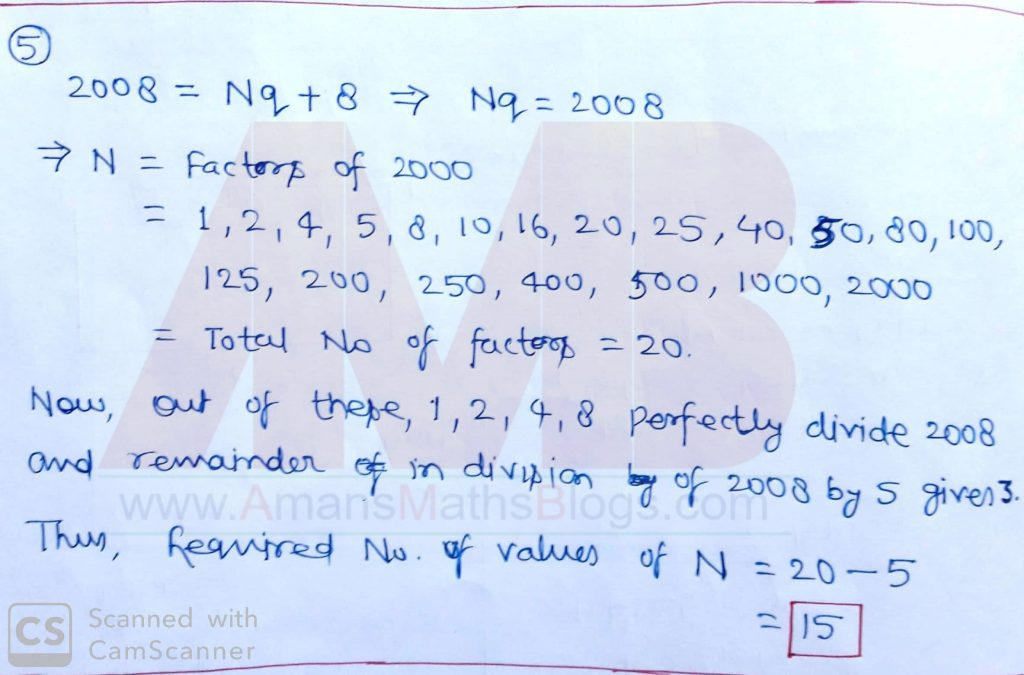NSEJS 2019-20 Maths Questions Bank With Solutions: Ques No 6

What is the product of all the roots of the equation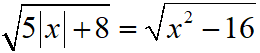?

Options:

A. -64

B. -24

C. 576

D. 24

Solution:NSEJS 2019-20 Maths Questions Bank With Solutions: Ques No 7

LCM of two numbers is 5775. Which of the following cannot be their HCF?

Options:

A. 175

B. 231

C. 385

D. 455

Solution: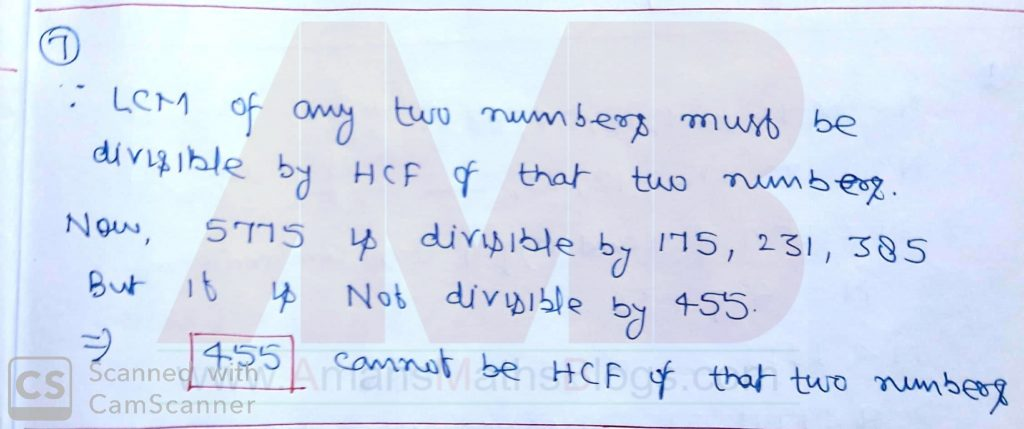NSEJS 2019-20 Maths Questions Bank With Solutions: Ques No 8

If a, b, c are distinct real numbers such that a + 1/b = b + 1/c = c + 1/a, evaluate abc.

Options:

A. ±√2

B. √2 – 1

C. √3

D. ±1

Solution:NSEJS 2019-20 Maths Questions Bank With Solutions: Ques No 9

If the equation (α2 – 5α + 6)x2 + (α2 – 3α + 2)x + (α2 – 4) = 0 has more than two roots, then the value of α is

Options:

A. 2

B. 3

C. 1

D. None of these

Solution:NSEJS 2019-20 Maths Questions Bank With Solutions: Ques No 10

Mr X with his eight children of different ages is on a family trip. His oldest child, who is 9 years old saw a license plate with a 4-digit number in which each of two digits appear two times. “Look daddy” she claims. “That number is evenly divisible by the age of each of us kids”. “That’s right” replies X “and the last two digits just happen to be my age”. Which of the following is not the age of one of Mr. X children?

Options:

A. 4

B. 5

C. 6

D. 7

Solution: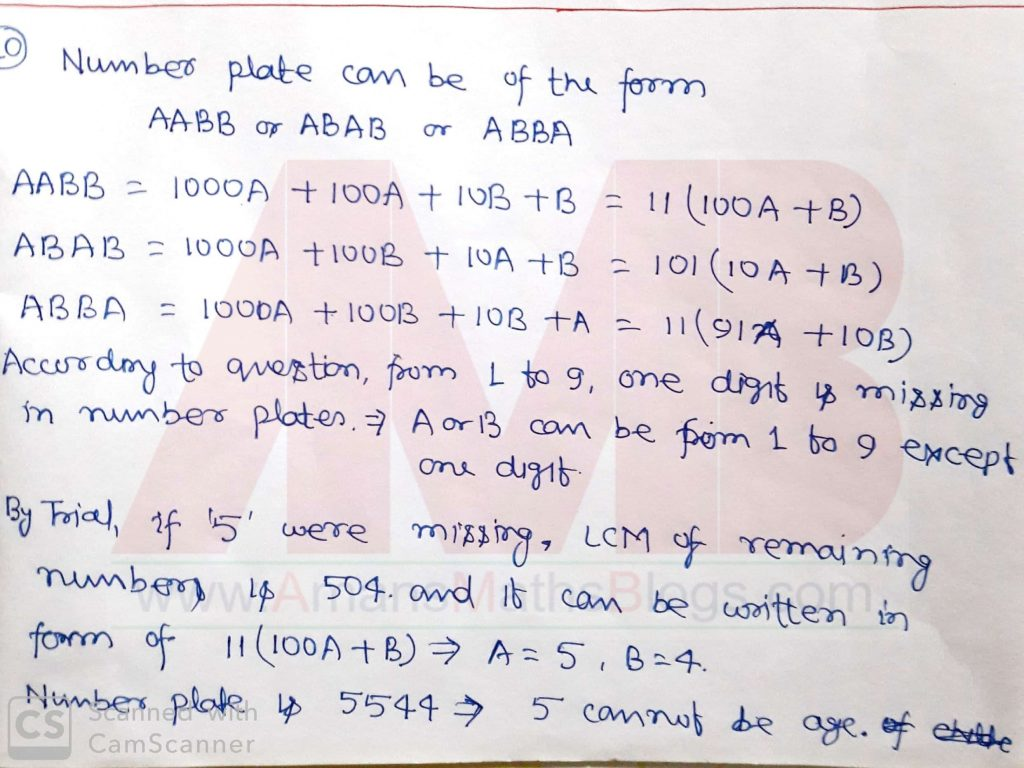NSEJS 2019-20 Maths Questions Bank With Solutions: Ques No 11

How many numbers lie between 11 and 1111 which divided by 9 leaves a remainder 6 and when divided by 21 leaves a remainder 12?

Options:

A. 18

B. 28

C. 8

D. None of these

Solution:NSEJS 2019-20 Maths Questions Bank With Solutions: Ques No 12

Two unbiased dice are rolled. What is the probability of getting a sum which is neither 7 nor 11?

Options:

A. 7/9

B. 7/18

C. 2/9

D. 11/18

Solution: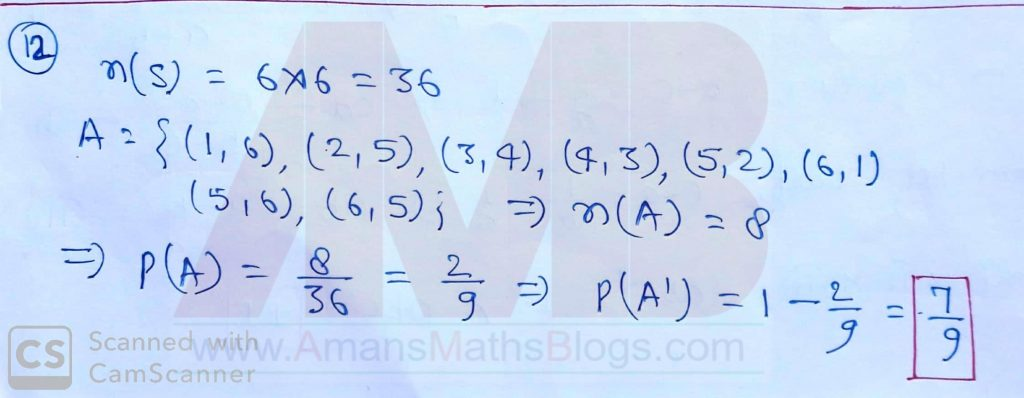NSEJS 2019-20 Maths Questions Bank With Solutions: Ques No 13

The solution of the equation 1 + 4 + 7 + … + x = 925 is

Options:

A. 73

B. 76

C. 70

D. 74

Solution:NSEJS 2019-20 Maths Questions Bank With Solutions: Ques No 14

If tanθ + secθ = 1.5, then the value of sinθ is

Options:

A. 5/13

B. 12/13

C. 3/5

D. 2/3

Solution: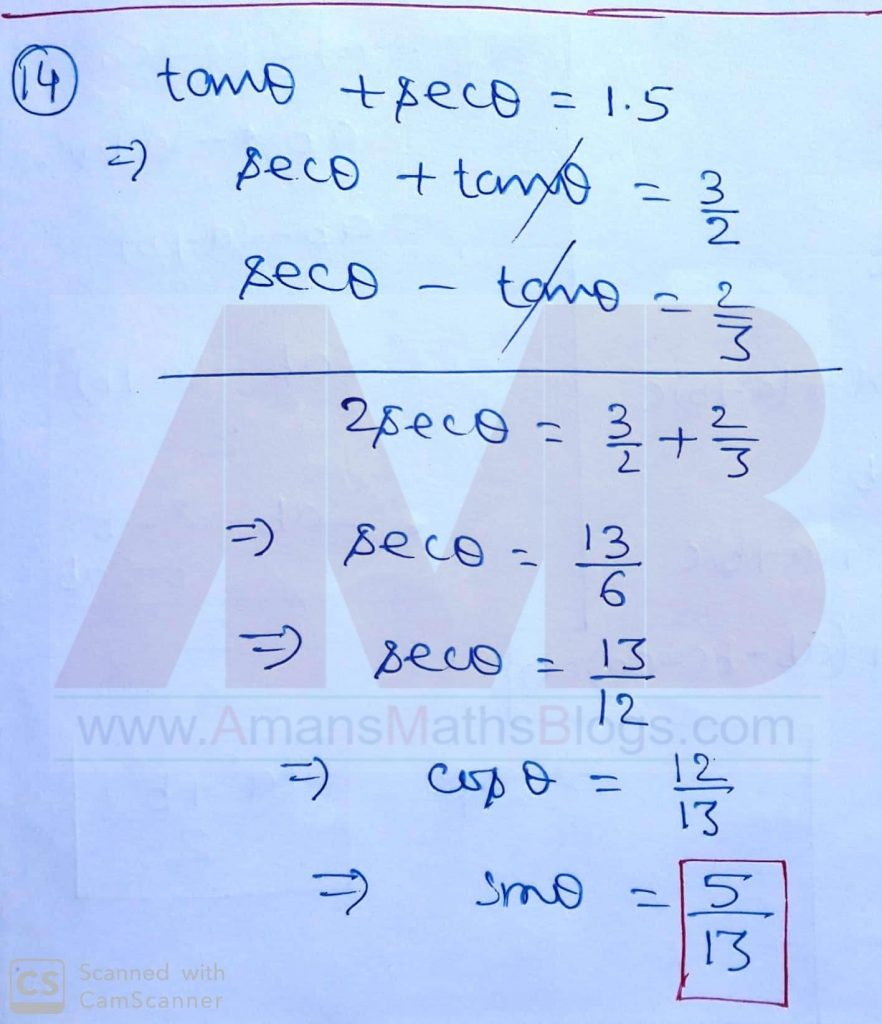NSEJS 2019-20 Maths Questions Bank With Solutions: Ques No 15

An observer standing at the top of a tower, find that the angle of elevation of a red bulb on the top of a light house of height H is α. Further, he finds that the angle of depression of reflection of the bulb in the ocean is β. Therefore, the height of the tower is

Options:

A. H(tanβ – tanα)/(tanβ + tanα)

B. Hsin(β – α)/cos(β + α)

C. H(cosα – cosβ)/(cotα + cotβ)

D. H

Solution: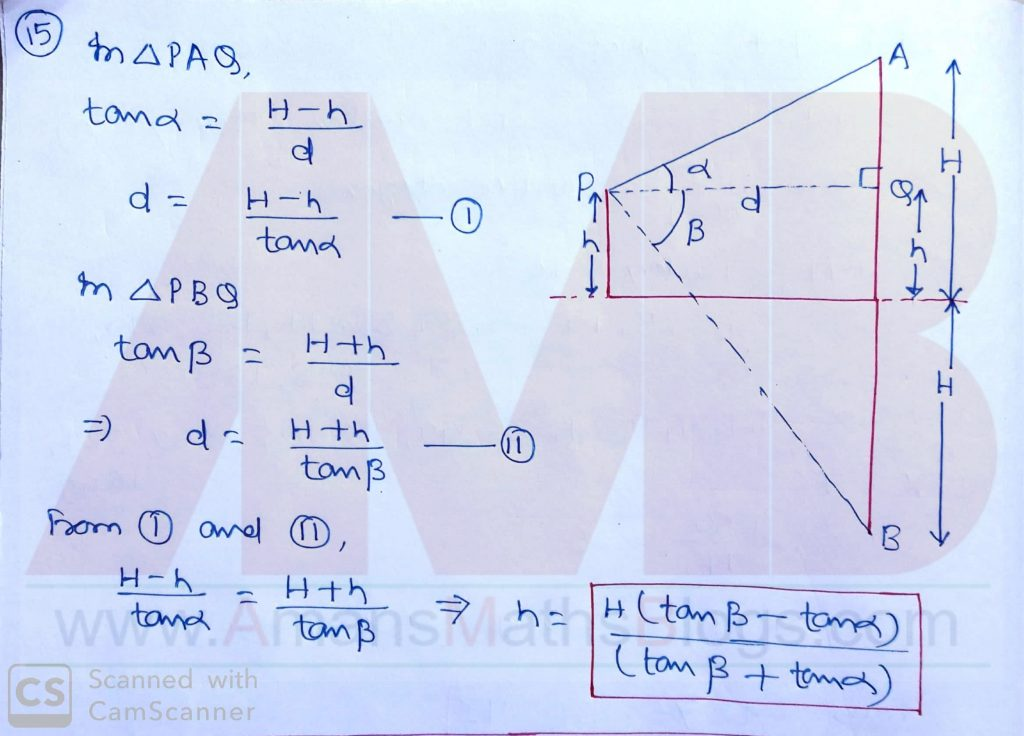NSEJS 2019-20 Maths Questions Bank With Solutions: Ques No 16

The sum of the roots of 1/(x + a) + 1/(x + b) = 1/c is zero. The product of roots is

Options:

A. 0

B. (a + b)/2

C. (-1/2)(a2 + b2)

D. 2(a2 + b2)

Solution: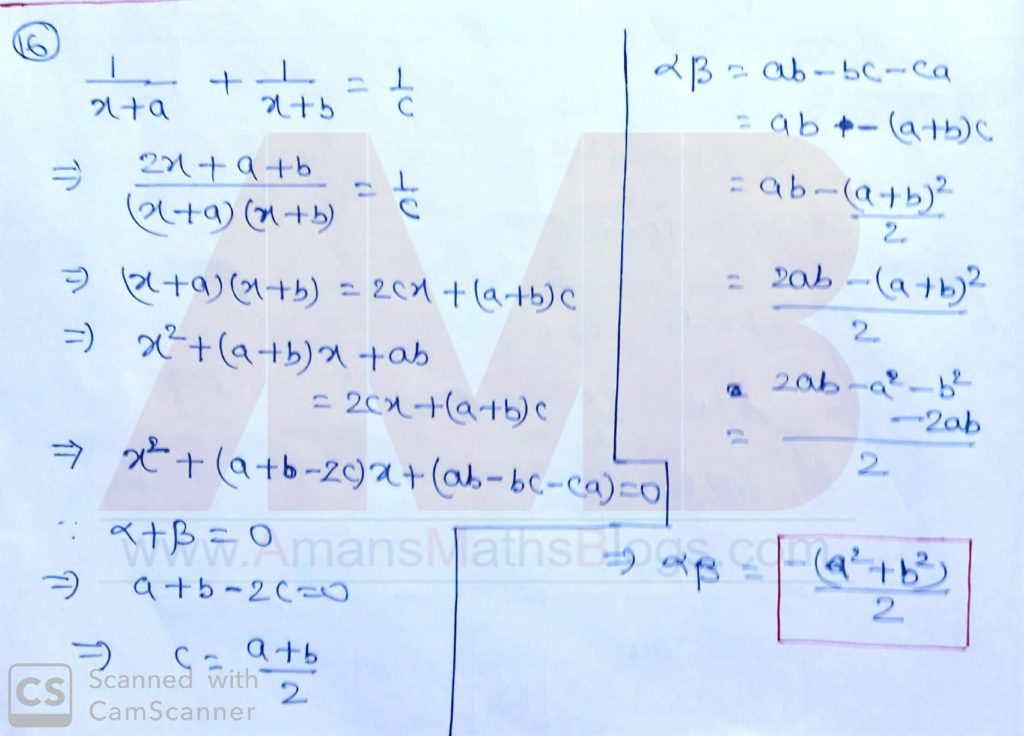NSEJS 2019-20 Maths Questions Bank With Solutions: Ques No 17

In a convex quadrilateral ABCD, the diagonals AC and BD meets at O and the measure of the angle AOB is 30 degree. If the areas of the triangle AOB, BOC, COD, DOA are 1, 2, 8 and 4 square units respectively, what is the product of the length of the diagonals AC and BD in square units?

Options:

A. 60

B. 56

C. 54

D. 64

Solution: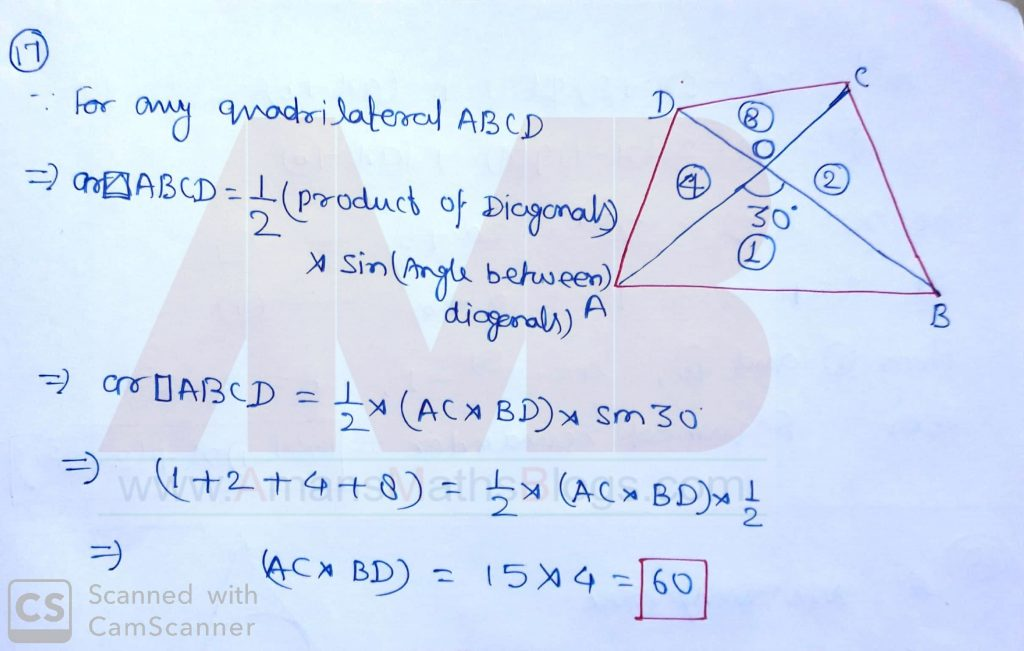NSEJS 2019-20 Maths Questions Bank With Solutions: Ques No 18

If sin2x + sin2y + sin2z = 0, then which of the following is NOT a possible value of cosx  + cosy + cosz?

Options:

A. 3

B. -3

C. 1

D. -2

Solution: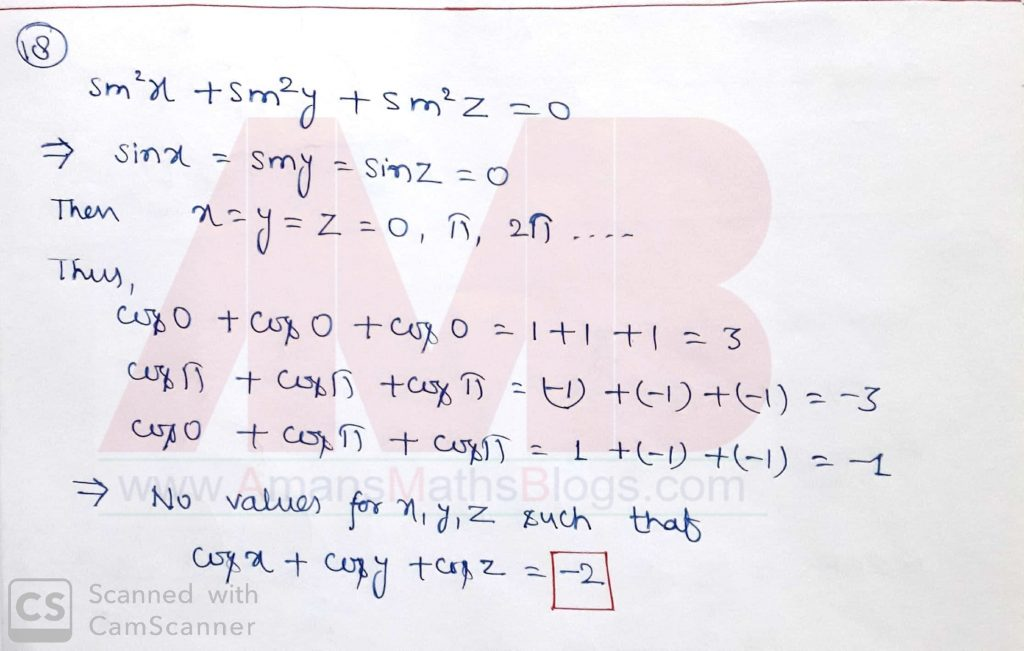NSEJS 2019-20 Maths Questions Bank With Solutions: Ques No 19

Find the remainder when x51 is divided by (x2 – 3x + 2).

Options:

A. x

B. (251 – 2)x + (2 – 251)

C. (251 – 1)x + (2 – 251)

D. 0

Solution:NSEJS 2019-20 Maths Questions Bank With Solutions: Ques No 20

In an equilateral triangle, three coins of radii 1 unit each are kept so that they touch each other and also sides of the triangle. The area of the triangle ABC (in square units) is

Options:

A. 4 + 2√3

B. 4√3 + 6

C. 12 + 7√3/4

D. 3 + 7√3/4

Solution: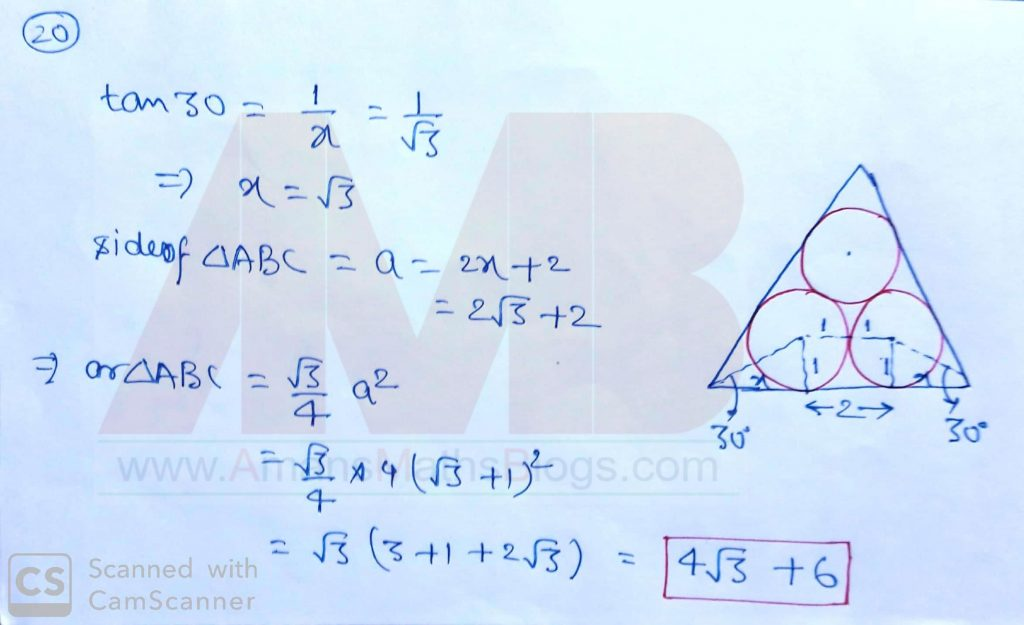For solution of NTSE 2019-20 Question Paper,

NSEJS
Previous Year Question Papers
2008-092009-102010-112011-122012-13
2013-142014-152015-162016-172017-18
2018-192019-20

## NSEJS Maths Questions Bank EBook

Get NSEJS 2008 to 2019 Maths Questions Bank with Solutions. To get this combo ebook, click below RED button.AMBiPi
error: Content is protected !!